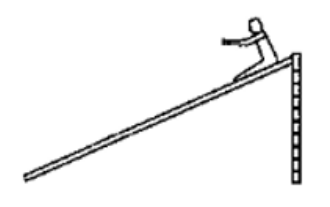# Solve the following :

Question:

In a children's park, there is a slide which has a total length of $10 \mathrm{~m}$ and a height of $8.0 \mathrm{~m}$ (figure 8E3). Vertical ladder are provided to reach the top. A boy weighing $200 \mathrm{~N}$ climbs up the ladder to the top of the slide and slides down to the ground. The average friction offered by the slide is three tenth of his weight. Find (a) the work done by the ladder on the boy as he goes up, (b) the work done by the slide on the boy as he comes down. Neglect any work done by forces inside the body of the boy.

Solution:(a) Work done, $W=0$ because no work is done by ladder.

(b) Work done $W=\mu R S=f d$

$=-(200 \times 3 / 10) \times 10=-600 \mathrm{~J}$

(c) Work done, $W=m g \sin \theta \times d=200 \times 8 / 10 \times 10$

$W=1600 \mathrm{~J}$